# PartialLinearFluid

Generic pure liquid model with constant cp, compressibility and thermal expansion coefficients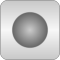# Package Contents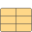ThermodynamicState A selection of variables that uniquely defines the thermodynamic state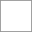BaseProperties Base properties of medium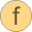setState_pTX Set the thermodynamic state record from p and T (X not needed)setState_phX Set the thermodynamic state record from p and h (X not needed)setState_psX Set the thermodynamic state record from p and s (X not needed)setState_dTX Set the thermodynamic state record from d and T (X not needed)setSmoothState Return thermodynamic state so that it smoothly approximates: if x > 0 then state_a else state_bpressure Return the pressure from the thermodynamic statetemperature Return the temperature from the thermodynamic statedensity Return the density from the thermodynamic statespecificEnthalpy Return the specific enthalpy from the thermodynamic statespecificEntropy Return the specific entropy from the thermodynamic statespecificInternalEnergy Return the specific internal energy from the thermodynamic statespecificGibbsEnergy Return specific Gibbs energy from the thermodynamic statespecificHelmholtzEnergy Return specific Helmholtz energy from the thermodynamic statevelocityOfSound Return velocity of sound from the thermodynamic stateisentropicExponent Return isentropic exponent from the thermodynamic stateisentropicEnthalpy Return isentropic enthalpyspecificHeatCapacityCp Return specific heat capacity at constant volumespecificHeatCapacityCv Return specific heat capacity at constant volume from the thermodynamic stateisothermalCompressibility Return the isothermal compressibility kappaisobaricExpansionCoefficient Return the isobaric expansion coefficientdensity_derp_h Return density derivative w.r.t. pressure at const specific enthalpydensity_derh_p Return density derivative w.r.t. specific enthalpy at constant pressuredensity_derp_T Return density derivative w.r.t. pressure at const temperaturedensity_derT_p Return density derivative w.r.t. temperature at constant pressuredensity_derX Returns the partial derivative of density with respect to mass fractions at constant pressure and temperaturemolarMass Return molar massT_ph Return temperature from pressure and specific enthalpyT_ps Return temperature from pressure and specific entropy

# Package Constants (28)

ThermoStates Value: Modelica.Media.Interfaces.Choices.IndependentVariables.pTX Type: IndependentVariables Description: Enumeration type for independent variables Value: "unusablePartialMedium" Type: String Description: Name of the medium Value: {mediumName} Type: String[:] Description: Names of the mixture substances. Set substanceNames={mediumName} if only one substance. Value: fill("", 0) Type: String[:] Description: Names of the additional (extra) transported properties. Set extraPropertiesNames=fill("",0) if unused Value: false Type: Boolean Description: = true, if u and d are not a function of pressure Value: true Type: Boolean Description: = true if medium contains the equation sum(X) = 1.0; set reducedX=true if only one substance (see docu for details) Value: true Type: Boolean Description: = true if medium contains the equation X = reference_X Value: 101325 Type: AbsolutePressure (Pa) Description: Reference pressure of Medium: default 1 atmosphere Value: 298.15 Type: Temperature (K) Description: Reference temperature of Medium: default 25 deg Celsius Value: fill(1 / nX, nX) Type: MassFraction[nX] (kg/kg) Description: Default mass fractions of medium Value: 101325 Type: AbsolutePressure (Pa) Description: Default value for pressure of medium (for initialization) Value: Modelica.SIunits.Conversions.from_degC(20) Type: Temperature (K) Description: Default value for temperature of medium (for initialization) Value: specificEnthalpy_pTX(p_default, T_default, X_default) Type: SpecificEnthalpy (J/kg) Description: Default value for specific enthalpy of medium (for initialization) Value: reference_X Type: MassFraction[nX] (kg/kg) Description: Default value for mass fractions of medium (for initialization) Value: fill(0, nC) Type: ExtraProperty[nC] Description: Default value for trace substances of medium (for initialization) Value: size(substanceNames, 1) Type: Integer Description: Number of substances Value: nS Type: Integer Description: Number of mass fractions Value: if fixedX then 0 else if reducedX then nS - 1 else nS Type: Integer Description: Number of structurally independent mass fractions (see docu for details) Value: size(extraPropertiesNames, 1) Type: Integer Description: Number of extra (outside of standard mass-balance) transported properties Value: 1.0e-6 * ones(nC) Type: Real[nC] Description: Default for the nominal values for the extra properties Value: Type: SpecificHeatCapacity (J/(kg·K)) Description: Specific heat capacity at constant pressure Value: Type: IsobaricExpansionCoefficient (¹/K) Description: Thermal expansion coefficient at constant pressure Value: Type: IsothermalCompressibility (¹/Pa) Description: Isothermal compressibility Value: Type: MolarMass (kg/mol) Description: Molar mass Value: Type: Density (kg/m³) Description: Density in reference conditions Value: Type: SpecificEnthalpy (J/kg) Description: Specific enthalpy in reference conditions Value: Type: SpecificEntropy (J/(kg·K)) Description: Specific entropy in reference conditions Value: Type: Boolean Description: If true, entries in thermodynamic Jacobian are constant, taken at reference conditions

# Information

This information is part of the Modelica Standard Library maintained by the Modelica Association.

#### Linear Compressibility Fluid Model

This linear compressibility fluid model is based on the assumptions that:

• The specific heat capacity at constant pressure (cp) is constant
• The isobaric expansion coefficient (beta) is constant
• The isothermal compressibility (kappa) is constant
• Pressure and temperature are used as states
• The influence of density on specific enthalpy (h), entropy (s), inner energy (u) and heat capacity (cv) at constant volume is neglected.

That means that the density is a linear function in temperature and in pressure. In order to define the complete model, a number of constant reference values are needed which are computed at the reference values of the states pressure p and temperature T. The model can be interpreted as a linearization of a full non-linear fluid model (but it is not linear in all thermodynamic coordinates). Reference values are needed for

1. the density (reference_d),
2. the specific enthalpy (reference_h),
3. the specific entropy (reference_s).

Apart from that, a user needs to define the molar mass, MM_const. Note that it is possible to define a fluid by computing the reference values from a full non-linear fluid model by computing the package constants using the standard functions defined in a fluid package (see example in liquids package).

In order to avoid numerical inversion of the temperature in the T_ph and T_ps functions, the density is always taken to be the reference density in the computation of h, s, u and cv. For liquids (and this model is intended only for liquids) the relative error of doing so is 1e-3 to 1e-4 at most. The model would be more "correct" based on the other assumptions, if occurrences of reference_d in the computations of h,s,u and cv would be replaced by a call to density(state). That would require a numerical solution for T_ps, while T_ph can be solved symbolically from a quadratic function. Errors from this approximation are small because liquid density varies little.

#### Efficiency considerations

One of the main reasons to use a simple, linear fluid model is to achieve high performance in simulations. There are a number of possible compromises and possibilities to improve performance. Some of them can be influenced by a flag. The following rules where used in this model:

• All forward evaluations (using the ThermodynamicState record as input) are exactly following the assumptions above.
• If the flag constantJacobian is set to true in the package, all functions that typically appear in thermodynamic Jacobians (specificHeatCapacityCv, density_derp_h, density_derh_p, density_derp_T, density_derT_p) are evaluated at reference conditions (that means using the reference density) instead of the density of the current pressure and temperature. This makes it possible to evaluate the thermodynamic Jacobian at compile time.
• For inverse functions using other inputs than the states (e.g pressure p and specific enthalpy h), the inversion is using the reference state whenever that is necessary to achieve a symbolic inversion.
• If constantJacobian is set to false, the above list of functions is computed exactly according to the above list of assumptions
Authors:
Francesco Casella
Dipartimento di Elettronica e Informazione
Politecnico di Milano
Via Ponzio 34/5
I-20133 Milano, Italy
email: casella@elet.polimi.it
and
Hubertus Tummescheit
Modelon AB
Ideon Science Park
SE-22730 Lund, Sweden
email: Hubertus.Tummescheit@Modelon.se

# Extended by (2)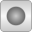LinearColdWater Modelica.Media.CompressibleLiquids Cold water model with linear compressibilityLinearWater_pT Modelica.Media.CompressibleLiquids.Common Base class for liquid, linear compressibility water models